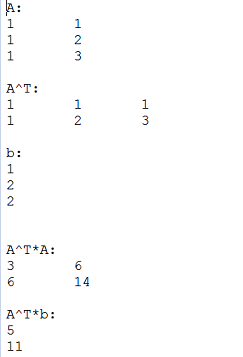2015年1月31日 星期六

[ RubyAlg ] MIT Linear Algebra, Spring 2005 - Lec16 (Linear Regression)

Source From HereRecallP 矩陣用來將 b 投影到其 Column Space 上, 因此考慮一下極端案例:
* b 就在 Column Space 上, 則 Pb = b
* b 與 Column Space 垂直, 則 Pb = 0

Projectionse = b - p = (b - Pb) = (I-P)b

Linear Regression(上面的 [1,2,3] 應該是 [1,2,2]1. require "alg/math/LinearAlgebra"
2.
3. LA = LinearAlgebra
4.
5. A = LA.newMtx3(3,2,[1,1,1,2,1,3])
6. b = LA.newMtx3(3,1,[1,2,2])
7. printf("A:\n%s\n", A)
8. printf("A^T:\n%s\n",A.t)
9. printf("b:\n%s\n", b)
10. printf("\n")
11.
12. A2 = A.t*A
13. b2 = A.t*b
14. printf("A^T*A:\n%s\n", A2)
15. printf("A^T*b:\n%s\n", b2)3C' + 6D' = 5
6C' + 14D' = 11

(此時的解 C'D' 與原先方程式的解 CD 是不同的!

1. x2 = A2.inv*b2
2. printf("[C',D']:\n%s\n", x2)

[C',D']:
0.6666666666666679
(C'=2/3)
0.5 (D'=1/2)(上面的 e1, e2, e3 還需乘與一個負號p 與 e 垂直: 很直覺, 因為 p 是在 Column Space, e 是在 Null Space of A^T 上, 這兩個分量注定垂直. (p*e=0)
e 與 Column Space 垂直: 這也是定義的觀點, 也就是說 e*[1,1,1] 與 e*[1,2,3] 皆會得到 zero!

Supplement
- If A has independent column vector(s), then A^T*A is invertable (Prove)
Suppose A^T*A*x=0, then x must be zero vector if A^T*A is invertable. Let's play a trick here. Consider:
x^T*A^T*A*x = (A*x)^T * (A*x)=0

So we can infer that A*x = 0, then we know that A has independent column which force x to be zero only!

2015年1月30日 星期五

[ GroovyGN ] Tuple Constructor Creation

Source From Here
Preface
Groovy 1.8 adds the @TupleConstructor annotation. With this annotation we can automatically create a tuple constructor at compile time. So the constructor can be found in the compiled class. For each property in the class a parameter in the constructor is created with a default value. The order of the properties defined in the class also defines the order of parameters in the constructor. Because the parameters have default values we can use Groovy syntax and leave parameters at the end of the parameter list out when we use the constructor.

Tuple Constructor Creation
If you run below code, you will raise Exception=groovy.lang.GroovyRuntimeException: Could not find matching constructor:
1. import groovy.transform.TupleConstructor
2.
3. //@TupleConstructor()
4. class Person {
5.     String name
6.     List likes
7.     private boolean active = false
8. }
9.
10. def person = new Person('mrhaki', ['Groovy''Java'])
11.
12. assert person.name == 'mrhaki'
13. assert person.likes == ['Groovy''Java']
14.
15. person = new Person('mrhaki')
16.
17. assert person.name == 'mrhaki'
18. assert !person.likes
If you uncomment the "@TupleConstructor", the code will run correctly!

We can also include fields as constructor parameters. We use the annotation attribute includeFields=true to activate this.
1. @TupleConstructor(includeFields=true)
2. class Person {
3.     String name
4.     List likes
5.     private boolean active = false
6.
7.     boolean isActivated() { active }
8. }
9.
10. def person = new Person('mrhaki', ['Groovy''Java'], true)
11.
12. assert person.name == 'mrhaki'
13. assert person.likes == ['Groovy''Java']
14. assert person.activated
15.
16. def classArgs = ["a", [], true]
17. def originalMapConstructor = Person.metaClass.retrieveConstructor(classArgs.toArray())
18. assert originalMapConstructor!=null
This time you can observe the input parameters of constructor including private field actived!

If we define our constructors in the class, then the @TupleConstructor annotation will not create extra constructors. But we can override this behaviour with the attribute value force=true. We have to make sure we don't have constructor conflicts ourselves, because now the annotation will create the extra constructors.
1. // use force attribute to force creation of constructor
2. // even if we define our own constructors.
3. import groovy.transform.TupleConstructor
4.
5. @TupleConstructor(force=true)
6. class Person {
7.     String name
8.     List likes
9.     private boolean active = false
10.
11.     // Our customized constructor
12.     Person(boolean active) {
13.         this.active = active
14.     }
15.
16.     boolean isActivated() { active }
17. }
18.
19. def person = new Person('mrhaki', ['Groovy''Java'])
20.
21. assert person.name == 'mrhaki'
22. assert person.likes == ['Groovy''Java']
23. assert !person.activated
24.
25. person = new Person(true)
26.
27. assert person.activated
If our class extends another class and we want to include the properties or fields of the super class we can use the attributes includeSuperProperties andincludeSuperFields. We can even instruct the annotation to create code in the constructor to call the super constructor of the super class with setting the annotation attribute callSuper=true to make this happen.
1. // include properties and fields from super class.
2. import groovy.transform.TupleConstructor
3.
4. @TupleConstructor(includeFields=true)
5. class Person {
6.     String name
7.     List likes
8.     private boolean active = false
9.
10.     boolean isActivated() { active }
11. }
12.
13. @TupleConstructor(callSuper=true, includeSuperProperties=true, includeSuperFields=true)
14. class Student extends Person {
15.     List courses
16. }
17.
18. def student = new Student('mrhaki', ['Groovy''Java'], true, ['IT'])
19.
20. assert student.name == 'mrhaki'
21. assert student.likes == ['Groovy''Java']
22. assert student.activated
23. assert student.courses == ['IT']
24.
25. printf("\t[Info] List all constructors of class Student:\n")
26. for(Constructor cstr in Student.class.getDeclaredConstructors())
27. {
28.     printf("\t%s\n", cstr)
29. }
Execution result:
[Info] List all constructors of class Student:
public Student()
public Student(java.lang.String)
public Student(java.lang.String,java.util.List)
public Student(java.lang.String,java.util.List,boolean)
public Student(java.lang.String,java.util.List,boolean,java.util.List)

Supplement
Overriding Groovy constructors
It’s rare to create parameterized constructors in Groovy since the Map constructor that’s added to all classes reduces the need for “real” constructors. There’sstill a case for defining constructors to enforce business rules, for example if an instance requires certain fields to be set in order for it to be valid. But in general we tend to just use the Map constructor...

Finding Constructors
A constructor declaration includes the name, modifiers, parameters, and list of throwable exceptions. The java.lang.reflect.Constructor class provides a way to obtain this information...

[ Python 常見問題 ] How to get symbolic link target in Python?

Source From  Here Question How do I extract the target path from a  symbolic link ? HowTo The problem with  os .readlink()  is it will onl...